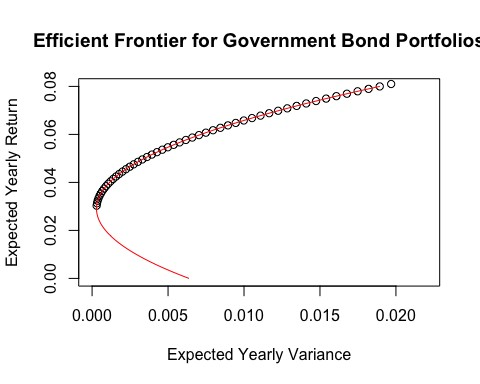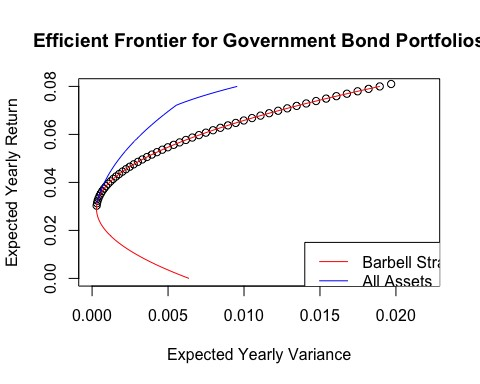# 第一部分

## 数字特征计算

pacman::p_load(fImport, PerformanceAnalytics, stringb, tidyverse)
# 将股票数据加载到一个时间序列对象的函数
importSeries = function(symbol,from,to) {
# 从雅虎读取金融数据
input = yahooSeries(symbol,from = from, to = to)
# 列名调整
inputReturn = symbol %.% ".Return"
CReturn = symbol %.% ".CReturn"
# 计算收益率并生成时间序列
colnames(input.Return) = inputReturn
input = merge(input,input.Return)
# 计算累积收益率并生成时间序列
FUN = function(x) log(x) - log(input.first))
colnames(input.CReturn) = CReturn
input = merge(input,input.CReturn)
# 删掉一些无用数据来释放内存
rm(input.first,
input.Return,
input.CReturn,
inputReturn,
CReturn)
# 返回时间序列
return(input)
}


# 获取短期、中期和长期政府债券的收益率序列
from = "2001-01-01"
to = "2011-12-16"
tlt = importSeries("tlt",from,to)
shy = importSeries("shy",from,to)
ief = importSeries("ief",from,to)
merged = merge(tlt,shy) %>% merge(ief)

vars = c("tlt.Return",
"shy.Return",
"ief.Return")
# 计算年化收益率 (t = table.AnnualizedReturns(merged[,vars], Rf = mean(merged[,"shy.Return"], na.rm=TRUE)))

##                  tlt.Return shy.Return ief.Return
## Annualized Return             0.0810     0.0303     0.0684
## Annualized Std Dev            0.1403     0.0173     0.0740
## Annualized Sharpe (Rf=3%)     0.3496    -0.0086     0.4974


## 杠铃策略

$$R_p=W_{TLT}*R_{TLT}+W_{SHY}*R_{SHY}$$

$$s.t. W_{TLT}+W_{SHY}=1$$

$$V_p=W_{TLT}^2*σ_{TLT}^2+W_{SHY}^2*σ_{SHY}^2+W_{TLT}*W_{SHY}*σ_{TLT}*σ_{SHY}*Corr\left(TLT,SHY\right)$$

$$s.t. W_{TLT}+W_{SHY}=1$$

## 风控模型

# 检查相关性
corr = cor(merged[,vars],use = "complete.obs")
c = corr["tlt.Return","shy.Return"]
# 假设一个杠铃策略是持有长期和短期资产
# 定义风险、收益
ws = NULL
wt = NULL
mu = NULL
sigma = NULL
# 50个观察 n=50
# 遍历杠铃策略的权重
rTLT = t["Annualized Return","tlt.Return"]
rSHY = t["Annualized Return","shy.Return"]
sTLT = t["Annualized Std Dev","tlt.Return"]
sSHY = t["Annualized Std Dev","shy.Return"]
for (i in 0:n){wsi = i/n;
wti = 1-wsi;
mui = wsi * rSHY + wti * rTLT
sigmai = wsi*wsi*sSHY*sSHY + wti*wti*sTLT*sTLT + wsi*wti*sSHY*sTLT*c
ws = c(ws,wsi)
wt = c(wt,wti)
mu = c(mu,mui)
sigma = c(sigma,sigmai) }
#风险收益的数据集
rrProfile = data.frame(ws=ws,wt=wt,mu=mu,sigma=sigma)


# 为模型拟合一个二次函数
fit = lm(rrProfile$sigma ~ rrProfile$mu + I(rrProfile$mu^2))  接下来，在图上添加拟合线。 # 得到回归系数 coe = fit$coefficients
# 得到每个回归预测的风险值
muf = NULL
sfit = NULL
for (i in seq(0,.08,by=.001)){
muf = c(muf,i)

s = coe + coe*i + coe*i^2
sfit = c(sfit,s)
}
# 绘图
plot(rrProfile$sigma, rrProfile$mu,
xlim=c(0,.022),
ylim=c(0,.08),
ylab="Expected Yearly Return",
xlab="Expected Yearly Variance",
main="Efficient Frontier for Government Bond Portfolios")
# 画出预测边值
lines(sfit,muf,col="red")tseries包中的portfolio.optim比较而言更好用。只需要输入预期收益率,该函数会直接返回出来最优组合权重。在最低预期收益率(比如 100% 持有 SHY)到最高预期收益率(比如100％持有TLT)之间修改输入的收益。注意，portfolio.optim会使用日收益率做计算，因此代码将不得不做一些处理并假设一年有255个交易日。

# 添加第三个标的
#除非想做一个格点搜索,否则就需要对每个级别的收益减少风险来优化投资组合。
# portfolio.optim 在时间序列中不能有 NA 值。

m2 = removeNA(merged[,vars])
wSHY = NULL
wIEF = NULL
wTLT = NULL
er = NULL
eStd = NULL
# 在收益水平之间不断循环搜索找到最优的投资组合，包括最小值(rSHY)和最大值(rTLT)
# portfolio.optim 使用日收益数据，因此不得不做出相应的调整
for (i in seq((rSHY+.001),(rTLT-.001),length.out=100)){
pm = 1+i
pm = log(pm)/255
opt = tseries::portfolio.optim(m2,pm=pm)
er = c(er,exp(pm*255)-1)
eStd = c(eStd,opt$ps*sqrt(255)) wTLT = c(wTLT,opt$pw)
wSHY = c(wSHY,opt$pw) wIEF = c(wIEF,opt$pw)
}
# 绘图
plot(rrProfile$sigma, rrProfile$mu,
xlim=c(0,.022),
ylim=c(0,.08),
ylab="Expected Yearly Return",
xlab="Expected Yearly Variance",
main="Efficient Frontier for Government Bond Portfolios")
# 画出预测边值
lines(sfit,muf,col="red")
# 画出三个标的的有效边界。
lines(eStd^2,er,col="blue")
legend(.014,0.015,c("Barbell Strategy","All Assets"),
col=c("red","blue"),
lty=c(1,1))
solution = data.frame(wTLT,wSHY,wIEF,er,eStd)


## 结论